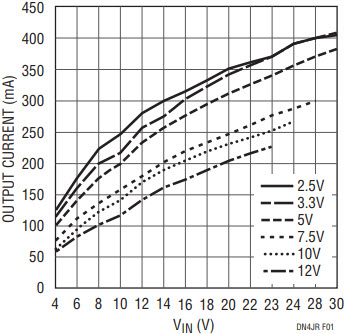# Increasing Output Voltage and Current Range Using Series-Connected Isolated μModule Converters

Linear Technology’s isolated µModule® converters are compact solutions for breaking ground loops. These converters employ a flyback architecture whose maximum output current varies with input voltage and output voltage. Although their output voltage range is limited to a maximum of 12V, one can increase the output voltage or the output current range. The solution simply involves connecting the secondary side of two or more isolated µModule converters in series.

The LTM8057 and LTM8058 UL60950-recognized 2kV AC isolated µModule converters will be used to demonstrate this design approach, which can also be applied to the LTM8046, LTM8047 and LTM8048. Let’s assume an output of 10V at 300mA is desired from a 20V input. Reviewing the maximum output current curve from Figure 1, we notice that a single LTM8057 is insufficient to meet the output current requirement under these conditions.Figure 1. Typical Maximum Output Current vs Input Voltage.

However, upon noticing that a single LTM8057 can deliver 300mA at 5V from a 20V input, a solution becomes apparent. Since the output voltage is isolated from the input, the outputs of two LTM8057s set at 5V can be connected in series to achieve a 10V output at 300mA (Figure 2).Figure 2. Two LTM8057 Modules with Outputs Connected in Series, Supporting a 10V, 300mA Output Application from 20VIN.

The same circuit in Figure 2 can also be used to increase the output voltage range when more than 12V is needed. By adjusting the feedback resistors to provide a 7.5V nominal output voltage, the combined output voltage has increased to 15V. The output current capability for the 15V is the same as that of the individual 7.5V modules (Figure 3).Figure 3. Two LTM8057 Modules with Outputs Connected in Series Deliver More Than 160mA at 15VOUT from 12VIN.

The circuit shown in Figure 2 supports a third option: providing positive and negative outputs with a common return. The return node for both outputs is the common connection in the middle of the output stack. With this approach the circuit in Figure 2 would have 5V and –5V outputs. Each output can be of different magnitude, since the output voltages for each converter are determined independently.

### Low Output Noise Series-Connected Converters

The low output spectrum noise advantage of the LTM8058 with its integrated LDO post regulator can still be maintained with series-connected outputs. Figure 4 shows the schematic for two LTM8058s with VOUT2, the output of the LDO connected in series for 10VOUT. Figures 5 and 6, respectively, show the output noise spectrum of the LTM8058 under a 100mA load at 10V with the LDO outputs connected in series (Figure 4 schematic) and the flyback outputs connected in series.Figure 4. Two LTM8058 Modules Connected with VOUT2 in Series for 10VOUT.Figure 5. Noise Spectrum for Two LTM8058s with the LDO Outputs Connected in Series Under a 100mA, 10VOUT Load.Figure 6. Noise Spectrum for Two LTM8058s with the Flyback Outputs Connected in Series Under a 100mA, 10VOUT Load.

Linear Technology’s isolated μModule converters provide a simple and compact solution for isolated power at regulated output voltages. The LTM8057 and LTM8058 successfully demonstrate that the output capabilities of isolated μModule converters can be increased by adding one or more isolated modules with the outputs tied in series while still preserving the output noise characteristics.